+ Text Only Site
+ Non-Flash Version
+ Contact GlennWing Area (Level 1) Answers What is the area of the Wright Brother's rectangular planform?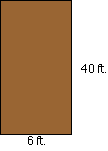Step 1: base = 6 ft.; height = 40 ft. Step 2: area of rectangle = b x h = 240 ft.2   What is the area of the F-18 (Blue Angels) trapezoidal planform?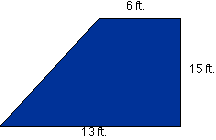Step 1: height = 15 ft.; base 1 = 13 ft.; base 2 = 6 ft. Step 2: area of trapezoid = ( h / 2 )( b1 + b2 ) = 142.5 ft.2 What is the area of the Concorde triangular planform? (Note: These are not the actual dimensions.)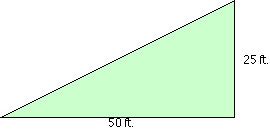Step 1: base = 50 ft.; height = 25 ft. Step 2: area of triangle = ( 1 / 2 ) x b x h = 625 ft.2   What is the area of the Space Shuttle's compound planform? (Note: These are not the actual dimensions.)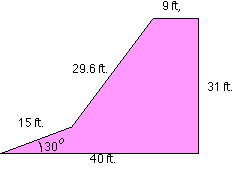Step 1: Divide the shape into three regions. From left to right, they are a triangle, trapezoid, and rectangle. Step 2: Region 1: base = 13 ft.; height = 7.5 ft. (Calculate the area of this triangle.) Region 2: height = 18 ft.; base1 = 31 ft.; base2 = 7.5 ft. (Calculate the area of this trapezoid.) Region 3: base = 9 ft; height = 31 ft (Calculate the area of this rectangle.) Step 3: Add the areas of the three regions. Total area = 674.3 ft.2   What is the area of the Boeing 747 trapezoidal planform? (Note: These are not the actual dimensions. The trapezoidal planform cannot be divided into two right triangles. Hint: Divide the figure into two scalene triangles and compute their areas.)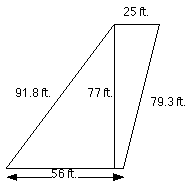Step 1: Divide the shape into three regions. From left to right, they are a triangle, rectangle, and triangle. The height of the first triangle is a straight line drawn down from the upper left-hand corner of the shape. The height of the second triangle is a straight line drawn up from the bottom right-hand corner of the shape. Step 2: Region 1: base = 50 ft. , height = 77 ft. (Calculate the area of this triangle.) Region 2: base = 6 ft. , height = 77 ft. (Calculate the area of this rectangle.) Region 3: base = 19 ft , height = 77 ft (Calculate the area of this triangle.) Step 3: Add the areas of the three regions. Total area = 3118.5 ft.2 Which of the wings has the largest area?  Boeing 747 Which of the wings has the smallest area?   F18 Design your own wing including its dimensions.  Answers will vary. What is the area of your airplane's planform?  Answers will vary. Explain why you designed your planform the way you did.  Answers will vary. List the wing types in order from the largest area to the smallest, including your own wing design.  Answers will vary

Related Pages:
Standards
Activity
Worksheet
Lesson Index
Aerodynamics Index+ Inspector General Hotline + Equal Employment Opportunity Data Posted Pursuant to the No Fear Act + Budgets, Strategic Plans and Accountability Reports + Freedom of Information Act + The President's Management Agenda + NASA Privacy Statement, Disclaimer, and Accessibility CertificationEditor: Tom Benson NASA Official: Tom Benson Last Updated: Thu, Jun 12 04:46:37 PM EDT 2014 + Contact Glenn